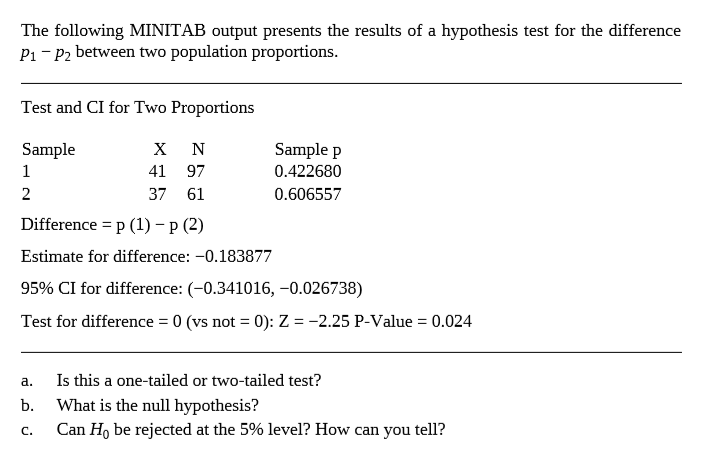# The following MINITAB output presents the results of a hypothesis test for the difference P1- P2 between two population proportions. Test and CI for Two Proportions Sample х N Sample p 41 97 0.422680 2 37 61 0.606557 Difference = p (1) – p (2) Estimate for difference: -0.183877 95% CI for difference: (-0.341016, -0.026738) Test for difference = 0 (vs not = 0): Z = -2.25 P-Value = 0.024 %3D %3D Is this a one-tailed or two-tailed test? a. b. What is the null hypothesis? Can Họ be rejected at the 5% level? How can you tell? C.

Questionhelp_outlineImage TranscriptioncloseThe following MINITAB output presents the results of a hypothesis test for the difference P1- P2 between two population proportions. Test and CI for Two Proportions Sample х N Sample p 41 97 0.422680 2 37 61 0.606557 Difference = p (1) – p (2) Estimate for difference: -0.183877 95% CI for difference: (-0.341016, -0.026738) Test for difference = 0 (vs not = 0): Z = -2.25 P-Value = 0.024 %3D %3D Is this a one-tailed or two-tailed test? a. b. What is the null hypothesis? Can Họ be rejected at the 5% level? How can you tell? C. fullscreen

## Expert Answer

### Want to see this answer and more?

Experts are waiting 24/7 to provide step-by-step solutions in as fast as 30 minutes!*

*Response times vary by subject and question complexity. Median response time is 34 minutes and may be longer for new subjects.
Tagged in© 2021 bartleby. All Rights Reserved.